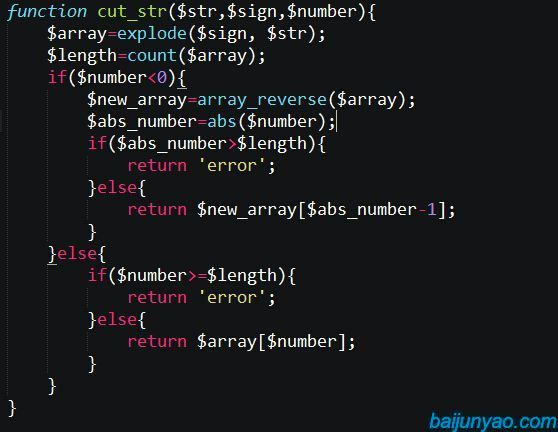PHP按符号截取字符串的指定部分

``\$str='123/456/789/abc';``

``echo substr(\$str,0,strpos(\$str, '/'))``
``或者``
``````\$array=explode('/', \$str);
echo \$array;
// 输出 123``````

``````echo substr(\$str,strpos(\$str,'/')+1);
//输出 456/789/abc``````

``echo trim(strrchr(\$str, '/'),'/');``
``或者如果知道斜杠的个数``
``````\$array=explode('/', \$str);
echo \$array;
//输出 abc``````

``````/**
* 按符号截取字符串的指定部分
* @param string \$str 需要截取的字符串
* @param string \$sign 需要截取的符号
* @param int \$number 如是正数以0为起点从左向右截  负数则从右向左截
* @return string 返回截取的内容
*/
function cut_str(\$str,\$sign,\$number){
\$array=explode(\$sign, \$str);
\$length=count(\$array);
if(\$number<0){
\$new_array=array_reverse(\$array);
\$abs_number=abs(\$number);
if(\$abs_number>\$length){
return 'error';
}else{
return \$new_array[\$abs_number-1];
}
}else{
if(\$number>=\$length){
return 'error';
}else{
return \$array[\$number];
}
}
}``````

``````echo cut_str(\$str,'/',0); //输出 123
echo cut_str(\$str,'/',2); //输出 789
echo cut_str(\$str,'/',-1);//输出 abc
echo cut_str(\$str,'/',-3);//输出 456``````